A cell is connected between the points A and C of a circular conductor ABCD of centre O with angle AOC = $60°$ . If ${\mathrm{B}}_{1}$ and ${\mathrm{B}}_{2}$ are the magnitudes of the magnetic fields at O due to the currents in ABC and ADC respectively, the ratio $\frac{{\mathrm{B}}_{1}}{{\mathrm{B}}_{2}}$ is: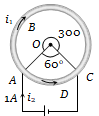1. 0.2

2. 6

3. 1

4. 5

Concept Questions :-

Magnetic field due to various cases
High Yielding Test Series + Question Bank - NEET 2020

Difficulty Level:

An electron, a proton, a deuteron and an alpha particle, each having the same speed are in a region of constant magnetic field perpendicular to the direction of the velocities of the particles. The radius of the circular orbits of these particles are respectively ${R}_{e}$, ${R}_{p}$${R}_{d}$ and ${R}_{\alpha }$. It follows that

(a) ${R}_{e}={R}_{p}$                (b) ${R}_{p}={R}_{d}$

(c) ${R}_{d}={R}_{\alpha }$                 (d) ${R}_{p}={R}_{\alpha }$

Concept Questions :-

Lorentz force
High Yielding Test Series + Question Bank - NEET 2020

Difficulty Level:

An infinitely long conductor PQR is bent to form a right angle as shown. A current I flows through PQR. The magnetic field due to this current at the point M is H1. Now another infinitely long straight conductor QS is connected at Q so that the current is I/2 in QR as well as in QS, The current in PQ remaining unchanged. The magnetic field at M is now ${H}_{\mathit{2}}$The ratio ${H}_{\mathit{1}}\mathit{/}{H}_{\mathit{2}}$ is given by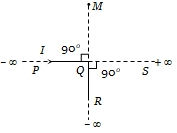(a) $\frac{1}{2}$

(b)  1

(c)  $\frac{2}{3}$

(d)  2

Concept Questions :-

Magnetic field due to various cases
High Yielding Test Series + Question Bank - NEET 2020

Difficulty Level:

A very long straight wire carries a current I. At the instant when a charge $+\underset{~}{O}$ at point P has velocity $\stackrel{\to }{v}$ , as shown, the force on the charge is: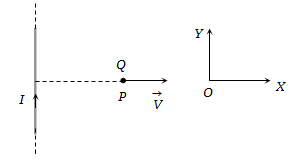1. Opposite to OX                            2. Along OX

3. Opposite to OY                             4. Along OY

Concept Questions :-

Lorentz force
High Yielding Test Series + Question Bank - NEET 2020

Difficulty Level:

An electric field of 1500 V / m and a magnetic field of 0.40 weber / $mete{r}^{2}$ act on a moving electron. The minimum uniform speed along a straight line the electron could have is

(a) $1.6×{10}^{15}m/s$                          (b) $6×{10}^{-16}m/s$

(c) $3.75×{10}^{3}m/s$                          (d) $3.75×{10}^{2}m/s$

Concept Questions :-

Lorentz force
High Yielding Test Series + Question Bank - NEET 2020

Difficulty Level:

A coil having N turns is wound tightly in the form of a spiral with inner and outer radii a and b respectively. When a current I passes through the coil, the magnetic field at the centre is:

1. $\frac{{\mathrm{\mu }}_{0}\mathrm{NI}}{\mathrm{b}}$                                  2. $\frac{2{\mathrm{\mu }}_{0}\mathrm{NI}}{\mathrm{a}}$

3. $\frac{{\mathrm{\mu }}_{0}\mathrm{NI}}{2\left(\mathrm{b}-\mathrm{a}\right)}\mathrm{ln}\frac{\mathrm{b}}{\mathrm{a}}$                         4. $\frac{{\mathrm{\mu }}_{0}{\mathrm{I}}^{\mathrm{N}}}{2\left(\mathrm{b}-\mathrm{a}\right)}\mathrm{ln}\frac{\mathrm{b}}{\mathrm{a}}$

Concept Questions :-

Magnetic field due to various cases
High Yielding Test Series + Question Bank - NEET 2020

Difficulty Level:

An electron, moving in a uniform magnetic field of induction of intensity $\stackrel{⇀}{B,}$ has its radius directly proportional to :

(a) Its charge                        (b) Magnetic field

(c) Speed                               (d) None of these

Concept Questions :-

Lorentz force
High Yielding Test Series + Question Bank - NEET 2020

Difficulty Level:

The magnetic field due to a straight conductor of uniform cross section of radius a and carrying a steady current is represented by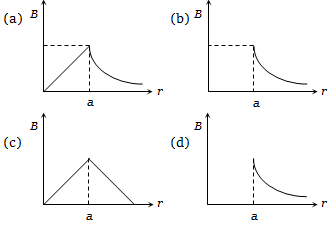Concept Questions :-

Magnetic field due to various cases
High Yielding Test Series + Question Bank - NEET 2020

Difficulty Level:

A non-planar loop of conducting wire carrying a current I is placed as shown in the figure. Each of the straight sections of the loop is of length 2a. The magnetic field due to this loop at the point P (a,0,a) points in the direction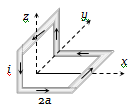(a)  $\frac{1}{\sqrt{2}}\left(-\stackrel{^}{\mathrm{j}}+\stackrel{^}{\mathrm{k}}\right)$                                   (c) $\frac{1}{\sqrt{3}}\left(\stackrel{^}{\mathrm{i}}+\stackrel{^}{\mathrm{j}}+\stackrel{^}{\mathrm{k}}\right)$

(b)  $\frac{1}{\sqrt{3}}\left(-\stackrel{^}{\mathrm{j}}+\stackrel{^}{\mathrm{k}}+\stackrel{^}{\mathrm{i}}\right)$                               (d)  $\frac{1}{\sqrt{2}}\left(\stackrel{^}{\mathrm{i}}+\stackrel{^}{\mathrm{k}}\right)$

Concept Questions :-

Magnetic field due to various cases
High Yielding Test Series + Question Bank - NEET 2020

Difficulty Level:

A particle of charge q and mass m is moving along the x-axis with a velocity v and enters a region of electric field E and magnetic field B as shown in the figure below. For which figure the net force on the charge may be zero?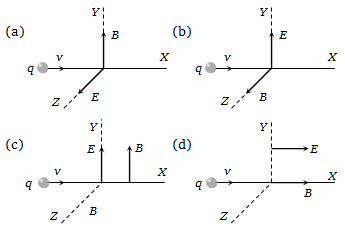Concept Questions :-

Lorentz force